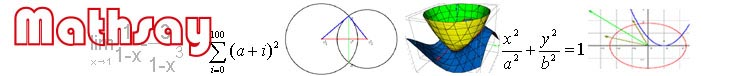Home

Support

Examples

Geometry

Trigonometry

Coordinate geometry

Function

Equation

Vector

3D geometry

3D plot

Limit & derivative

Math symbol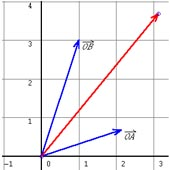Plot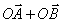.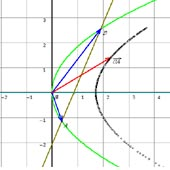y2=2px, a straight line passes its focus and intersects it in A and B. Make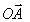and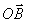. Show the trace of.